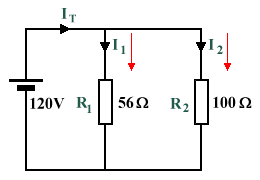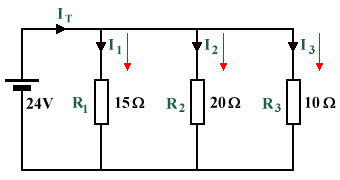# Answers to Problems - The Current Divider

1. In the circuit illustrated below, two resistors are in parallel with a 120 V supply voltage. If the resistance values of R1 and R2 are 56 Ω and 100 Ω respectively, find the current flowing through each resistor (I1 and I2), the total current flowing through the circuit (IT), and the total circuit resistance (RT).A current divider consisting of two resistors in parallel with a supply voltage

 I1  = 120 V =  2.143 A   (Ohm's law) 56 Ω

 I2  = 120 V =  1.2 A   (Ohm's law) 100 Ω

IT  =  2.143 A  +  1.2 A  =  3.343 A   (Kirchhoff's current law)

 RT  = 120 V =  35.896 Ω   (Ohm's law) 3.343 A

2. In the circuit illustrated below, three resistors are in parallel with a 24 V supply voltage. If the resistance values of R1, R2 and R3 are 15 Ω, 20 Ω and 10 Ω respectively, find the current flowing through each resistor (I1, I2 and I3), the total current flowing through the circuit (IT), and the total circuit resistance (RT).A current divider consisting of three resistors in parallel with a supply voltage

 I1  = 24 V =  1.6 A   (Ohm's law) 15 Ω

 I2  = 24 V =  1.2 A   (Ohm's law) 20 Ω

 I3  = 24 V =  2.4 A   (Ohm's law) 10 Ω

IT  =  1.6 A  +  1.2 A  +  2.4 A  =  5.2 A   (Kirchhoff's current law)

 RT  = 24 V =  4.615 Ω   (Ohm's law) 5.2 A